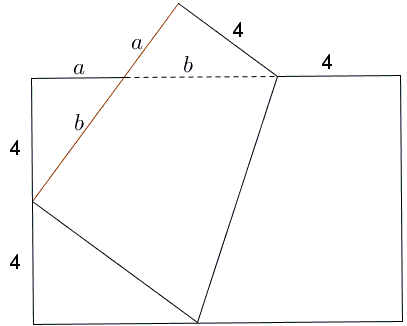# Egyptian Triangle By Paper Folding II

A rectangular sheet of paper is folded so that one corner bisects the shorter side. Triangles I and II are congruent. If the length of the shorter side is $8,$ find the length of the longer side.This is a problem from a 2011 olympiad for the seventh (the USA eighth) grade at the Moscow State University.

Solution

### References

1. I. Yashchenko, Invitation to a Mathematical Festival, MSRI/AMS, 2013Paper Folding GeometryA rectangular sheet of paper is folded so that one corner bisects the shorter side. Triangles I and II are congruent. If the length of the shorter side is 8, find the length of the longer side.It's convenient to introduce segment lengths $a$ and $b,$ as in the diagram. The folded short side of the rectangle is $a+b=8$ so that the long side is $a+b+4=12.$

The right triangles I and II are of interest in their own right. Each has one leg equal to $4$ and the sum of the other leg and hypotenuse $8.$ You'd guess it straight away what triangle it is, but to make sure let's use the Pythagorean theorem:

$4^{2}+a^{2}=(8-a)^2,$

which leads to $16=64-16a,$ or $a=3$, implying the famous Egyptian 3-4-5 triangle. The dimensions of the rectangle - $8\times 12$ - suggest an easy paper-folding construction of such a triangle which would start with first getting a not too wide a piece of paper, folding a square and then getting two more, which would lead to a rectangle of relative dimensions $2\times 3.$